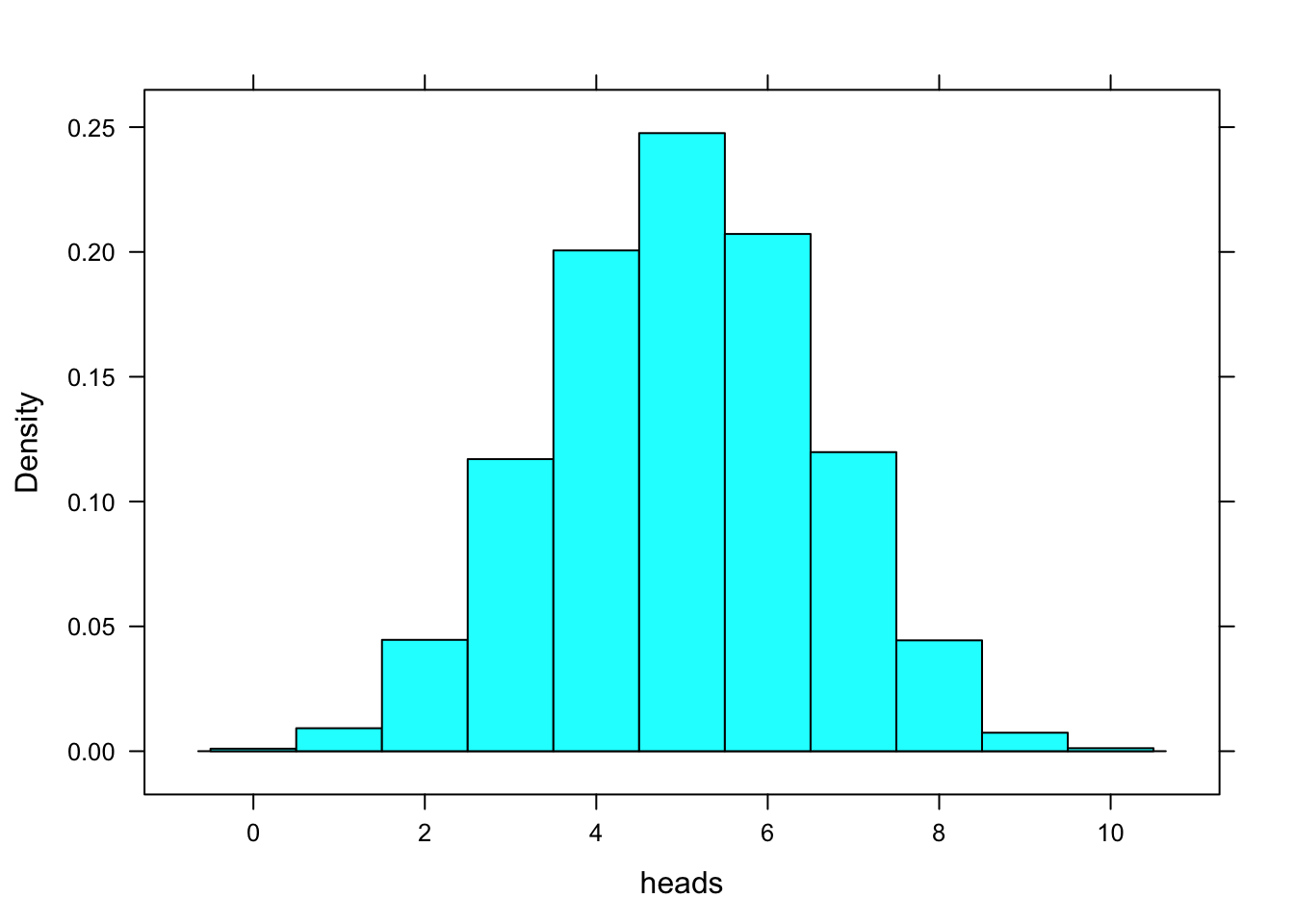library(mosaic)

## Bayes Rule

In Chapter 5, Cartwright refers to Bayes’ Rule. Let’s introduce Bayes’ Rule as a function in R. We need a function of the probability that the outcome is true given the “signal” $$\theta$$, or, phrased differently, given some “data” (often called D.) Therefore, we’re looking for something like:

$Pr(\mbox{Outcome} | \mbox{signal} = \frac{\theta p}{\theta p - (1 - \theta)(1 - p)}$

bayesrule <- function(p, theta) {
(theta * p) / (theta * p + ( 1 - theta)*(1 - p))
}

Now that we have a function called bayesrule(), we can specify values for theta and p in order to get bayesrule() to take the values of theta and p as arguments. Let’s use the values Cartwright initially suggests:

theta <- 0.9
p <- 0.4
bayesrule(p, theta)
##  0.8571429

If we wanted to, we could substitute in new values for theta and p, as below:

theta <- 0.7
p <- 0.5
bayesrule(p, theta)
##  0.7

Notice how when p = 0.5, the probability that the outcome is true is simply the accuracy of the signal (here 0.7). So when you “trust” your friend 70% and you are fifty-fifty in your preferences, you’ll be 70% likely to go with what your friend suggests untill you encounter a new signal (or new data).

## Models of Choice with uncertainty

Let’s put together a

ufn1 <- function(w){
(w)^(0.5)
}
wealth <- 0
winnings <- 2
u1 <- ufn1(wealth + winnings)
u2 <- ufn1(wealth)
p <- 0.6
exp.u <- function(p, u1, u2) {
p*u1 + (1 - p)*(u2)
}
exp.u(p, u1, u2)
##  0.8485281

## Gambler’s Fallacy

The gambler’s fallacy: we do not expect many heads (or tails) in a row, even though that could occur totally randomly

c1 <- rflip(50)
c1
##
## Flipping 50 coins [ Prob(Heads) = 0.5 ] ...
##
## T T T T H T H T T T T T H T T H H H H H T T T H T H T H T H T T H
## H H T T T H T H H T H H H T H H T
##
## Number of Heads: 23 [Proportion Heads: 0.46]
cartwright2 <- data.frame(c("H", "T", "T", "H", "H", "H", "T", "T", "H", "T", "T", "H", "T", "H", "H", "T", "H", "T", "T", "T", "H", "H", "T", "H", "H", "H", "T", "H", "T", "T", "H", "H", "T", "H", "T", "T", "T", "H", "H", "T", "H", "H", "T", "T", "T", "H", "T", "H", "H", "T"))
cartwright2
##    heads
## 1      H
## 2      T
## 3      T
## 4      H
## 5      H
## 6      H
## 7      T
## 8      T
## 9      H
## 10     T
## 11     T
## 12     H
## 13     T
## 14     H
## 15     H
## 16     T
## 17     H
## 18     T
## 19     T
## 20     T
## 21     H
## 22     H
## 23     T
## 24     H
## 25     H
## 26     H
## 27     T
## 28     H
## 29     T
## 30     T
## 31     H
## 32     H
## 33     T
## 34     H
## 35     T
## 36     T
## 37     T
## 38     H
## 39     H
## 40     T
## 41     H
## 42     H
## 43     T
## 44     T
## 45     T
## 46     H
## 47     T
## 48     H
## 49     H
## 50     T
tally(~ heads, data = cartwright2)
##
##  H  T
## 25 25
c3 <- rflip(50)
c3
##
## Flipping 50 coins [ Prob(Heads) = 0.5 ] ...
##
## H T T T H H H H H H T H T T T T H H T H T H H H T T H H T H T H H
## T T H T T T T H T H H H T H H T H
##
## Number of Heads: 27 [Proportion Heads: 0.54]
GamblersFal <- do(5000) * rflip(10)
histogram( ~ heads, data = GamblersFal, width = 1 )## Checking values of Bayes Rule in a Table

I didn’t end up using this. Ignore it. I’m keeping it here for my notes later.

theta.tab <- seq(0.1, 1, by = 0.1)
p.tab <- seq(0.1, 1, by = 0.1)
ptheta <- data.frame(theta.tab, p.tab)